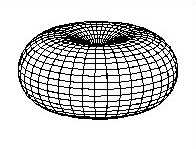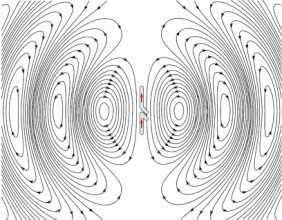Select PageThe radiation pattern of a simple omnidirectional antenna, a vertical half-wave dipole antenna. In this graph the antenna is at the center of the “donut,” or torus. Radial distance from the center represents the power radiated in that direction. The power radiated is maximum in horizontal directions, dropping to zero directly above and below the antenna.Animation of an omnidirectional half-wave dipole antenna transmitting radio waves. The antenna in the center is two vertical metal rods, with an alternating current applied at its center from a radio transmitter (not shown). Loops of electric field (black lines) leave the antenna and travel away at the speed of light; these are the radio waves. This diagram only shows the radiation in a single plane through the axis, the radiation is symmetrical about the antenna’s vertical axis.

## Types

Common types of low-gain omnidirectional antennas are the whip antenna, “Rubber Ducky” antenna, ground plane antenna, vertically oriented dipole antenna, discone antenna, mast radiator, horizontal loop antenna (sometimes known colloquially as a ‘circular aerial’ because of the shape) and the halo antenna.

Higher-gain omnidirectional antennas can also be built. “Higher gain” in this case means that the antenna radiates less energy at higher and lower elevation angles and more in the horizontal directions. High-gain omnidirectional antennas are generally realized using collinear dipole arrays. These consist of multiple half-wave dipoles mounted collinearly (in a line), fed in phase.  The coaxial collinear (COCO) antenna uses transposed coaxial sections to produce in-phase half-wavelength radiators.  A Franklin Array uses short U-shaped half-wavelength sections whose radiation cancels in the far-field to bring each half-wavelength dipole section into equal phase. Another type is the Omnidirectional Microstrip Antenna (OMA).

## Analysis

Omnidirectional radiation patterns are produced by the simplest practical antennas, monopole and dipole antennas, consisting of one or two straight rod conductors on a common axis. Antenna gain (G) is defined as antenna efficiency (e) multiplied by antenna directivity (D) which is expressed mathematically as:
$G=eD$. A useful relationship between omnidirectional radiation pattern directivity (D) in decibels and half-power beamwidth (HPBW) based on the assumption of a
$\sin(b\theta )/{b\theta }$pattern shape is:

$D\approx 10\log _{10}\left({\frac {101.5}{{\text{HPBW}}-0.00272\,{\text{HPBW}}^{2}}}\right)\ {\text{ dB}}.$error: Content is protected !!